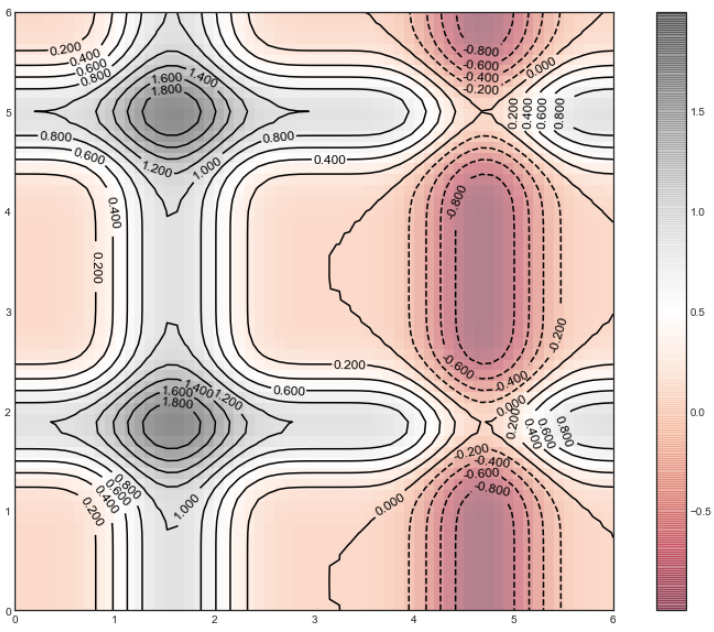# Generate Contour Plots Using Python’s MatplotlibGenerate Contour Plots Using Python’s Matplotlib. A simple and easy tutorial on making Contour plots

I am sure you have seen a contour plot before. At least in weather data. It is used a lot in presenting meteorological or geographical data. But it is also used to present density, brightness, and electric potential. It is used a lot in data analysis and machine learning. It shows how a response variable relates to two predictor variables.

It gives a two-dimensional view where all the points having the same response are connected by a line. That line is a contour line.

Three main elements of a contour plot:

1. x-axis and the y-axis shows the predictors
2. Contour lines to represent the same response values
3. Colored bands that provide a range of response values

### Here is how to draw a contour plot

First import the necessary packages.

``````import numpy as np
import matplotlib.pyplot as plt
import pylab``````

Let’s generate some x and y values

``````xp = np.arange(-8, 10, 2)
yp = np.arange(-8, 10, 2)``````

You can probably imagine how ‘xp’ and ‘yp’ look like. But still, just have a look.

``xp``

Output:

``array([-8, -6, -4, -2,  0,  2,  4,  6,  8])``

Input:

``yp``

Output:

``````array([-8, -6, -4, -2,  0,  2,  4,  6,  8])
``````

## Data Science Projects | Data Science | Machine Learning | Python

Practice your skills in Data Science with Python, by learning and then trying all these hands-on, interactive projects, that I have posted for you.

## Data Science Projects | Data Science | Machine Learning | Python

Practice your skills in Data Science with Python, by learning and then trying all these hands-on, interactive projects, that I have posted for you.

## Data Science Projects | Data Science | Machine Learning | Python

Practice your skills in Data Science with Python, by learning and then trying all these hands-on, interactive projects, that I have posted for you.

## Data Science Projects | Data Science | Machine Learning | Python

Practice your skills in Data Science with Python, by learning and then trying all these hands-on, interactive projects, that I have posted for you.

## Data Science Projects | Data Science | Machine Learning | Python

Practice your skills in Data Science with Python, by learning and then trying all these hands-on, interactive projects, that I have posted for you.